# PROJECTIONS OF SOLIDS Definition of Solid A solid

• Slides: 41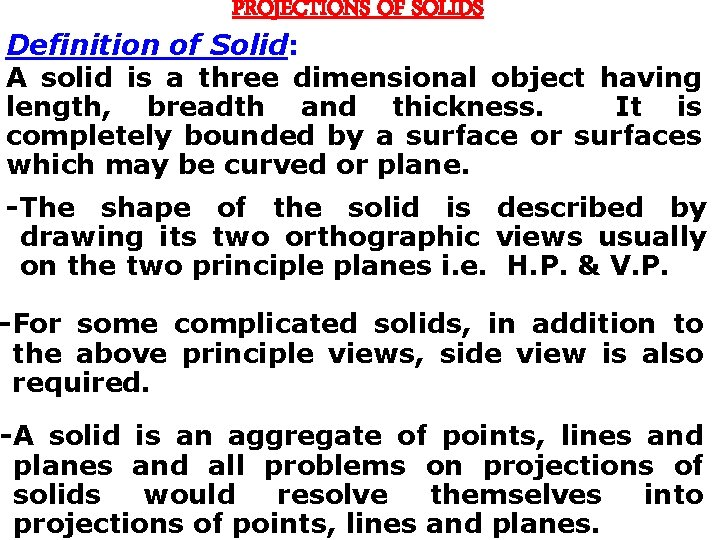PROJECTIONS OF SOLIDS Definition of Solid: A solid is a three dimensional object having length, breadth and thickness. It is completely bounded by a surface or surfaces which may be curved or plane. -The shape of the solid is described by drawing its two orthographic views usually on the two principle planes i. e. H. P. & V. P. -For some complicated solids, in addition to the above principle views, side view is also required. -A solid is an aggregate of points, lines and planes and all problems on projections of solids would resolve themselves into projections of points, lines and planes.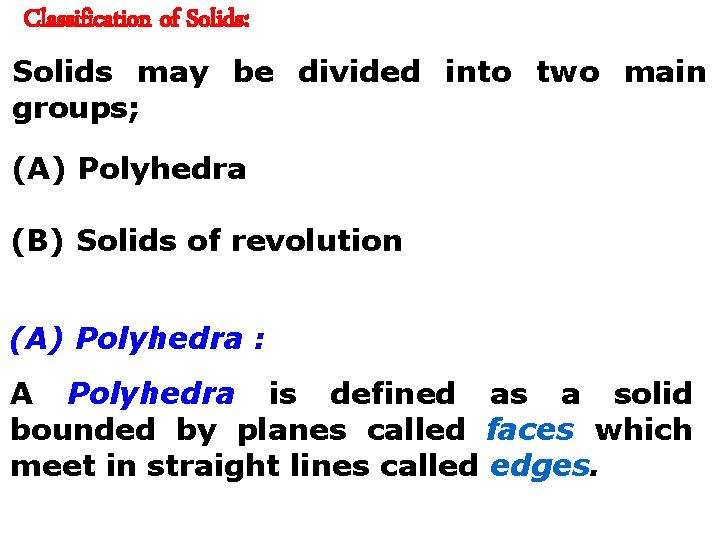Classification of Solids: Solids may be divided into two main groups; (A) Polyhedra (B) Solids of revolution (A) Polyhedra : A Polyhedra is defined as a solid bounded by planes called faces which meet in straight lines called edges.There are seven regular Polyhedra which may be defined as stated below; (1) Prism (2) Pyramid (3) Tetrahedron (4) Cube or Hexahedron: (5) Octahedron: (6) Dodecahedron: (7) Icosahedron: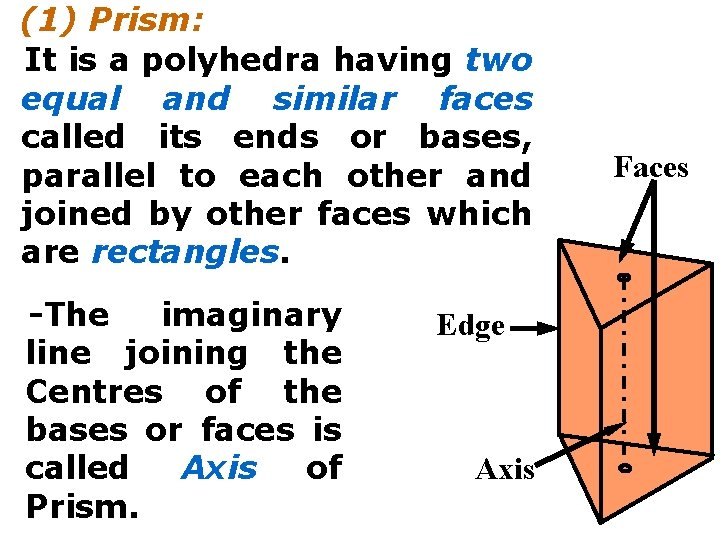(1) Prism: It is a polyhedra having two equal and similar faces called its ends or bases, parallel to each other and joined by other faces which are rectangles. -The imaginary line joining the Centres of the bases or faces is called Axis of Prism. Edge Axis Faces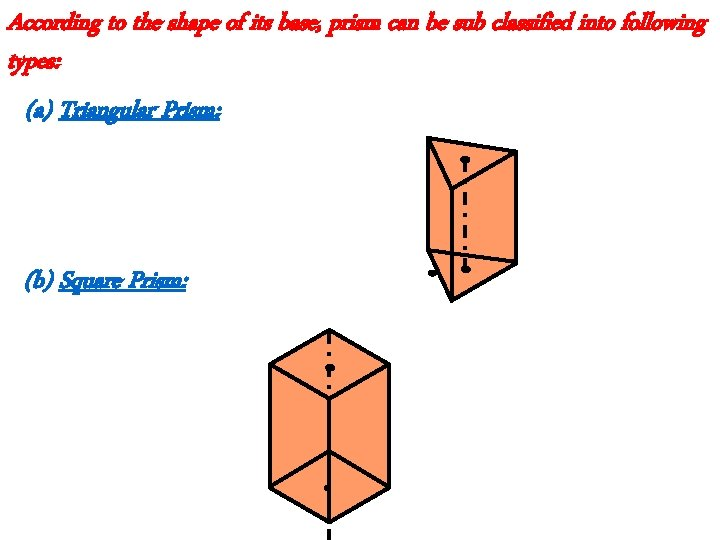According to the shape of its base, prism can be sub classified into following types: (a) Triangular Prism: (b) Square Prism:(c) Pentagonal Prism: (d) Hexagonal Prism:(2) Pyramid: This is a polyhedra having plane surface as a base and a number of triangular faces meeting at a point called the Vertex or Apex. -The imaginary line joining the Apex with the Centre of the base is called Axis of pyramid. Edge Axis BaseAccording to the shape of its base, pyramid can be sub classified into following types: (a) Triangular Pyramid: (b) Square Pyramid:(c) Pentagonal Pyramid: (d) Hexagonal Pyramid:(B) Solids of Revolutions: When a solid is generated by revolutions of a plane figure about a fixed line (Axis) then such solids are named as solids of revolution. Solids of revolutions may be of following types; (1) Cylinder (2) Cone (3) Sphere (4) Ellipsoid (5) Paraboloid (6) Hyperboloid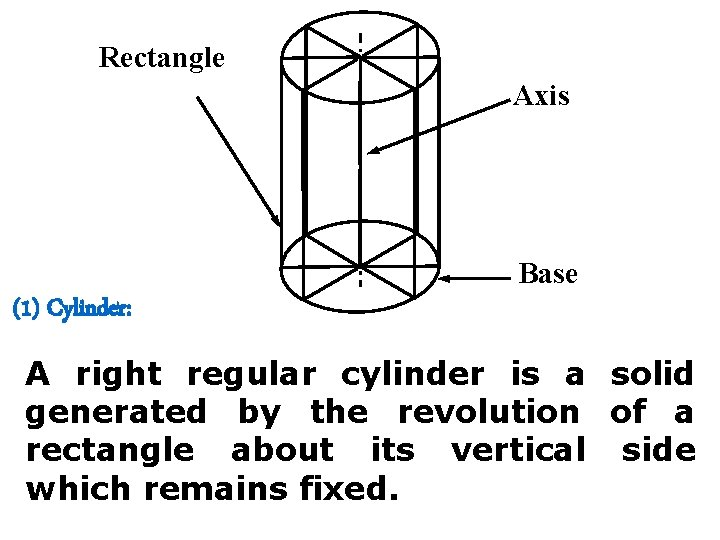Rectangle Axis Base (1) Cylinder: A right regular cylinder is a solid generated by the revolution of a rectangle about its vertical side which remains fixed.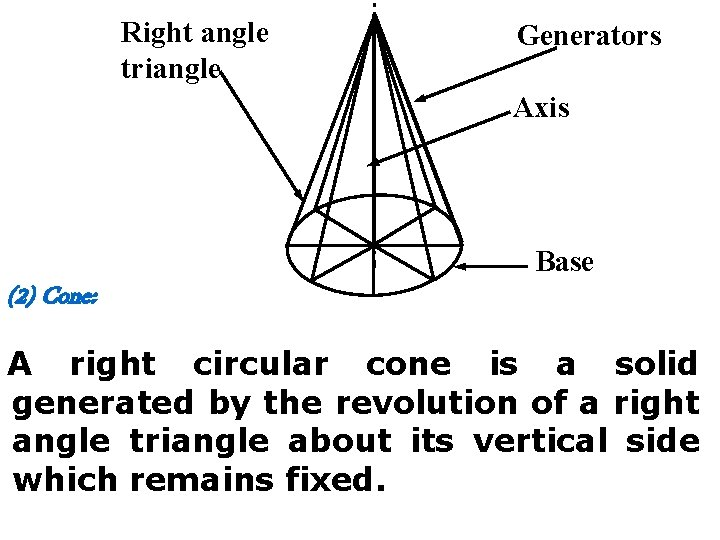Right angle triangle Generators Axis Base (2) Cone: A right circular cone is a solid generated by the revolution of a right angle triangle about its vertical side which remains fixed.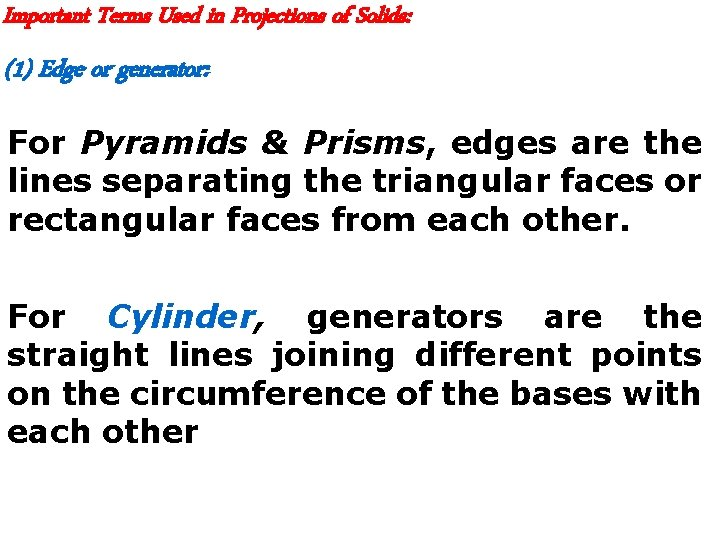Important Terms Used in Projections of Solids: (1) Edge or generator: For Pyramids & Prisms, edges are the lines separating the triangular faces or rectangular faces from each other. For Cylinder, generators are the straight lines joining different points on the circumference of the bases with each other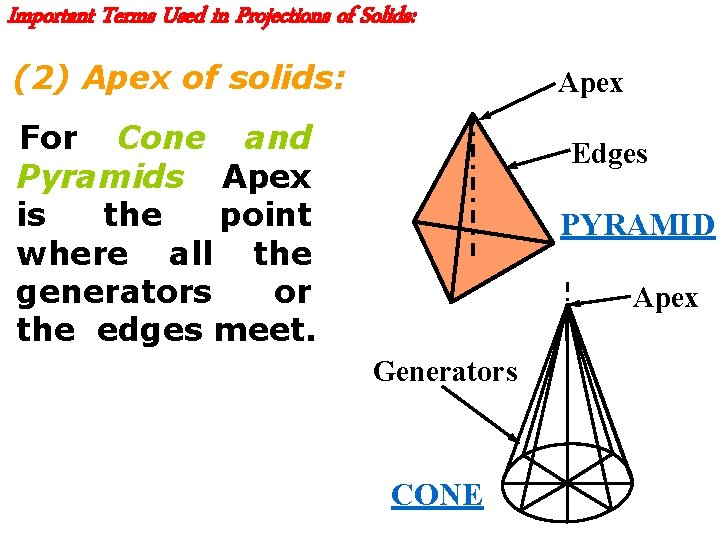Important Terms Used in Projections of Solids: (2) Apex of solids: Apex For Cone and Pyramids, Apex is the point where all the generators or the edges meet. Edges PYRAMID Apex Generators CONERectangle Axis Generators Base Edge CYLINDER PRISM Axis FacesImportant Terms Used in Projections of Solids: (3) Axis of Solid: For Cone and Pyramids, Axis is an imaginary line joining centre of the base to the Apex. For Cylinder and Prism, Axis is an imaginary line joining centres of ends or bases.Important Terms Used in Projections of Solids: (4) Right Solid: A solid is said to be a Right Solid if its axis is perpendicular to its base. Axis Base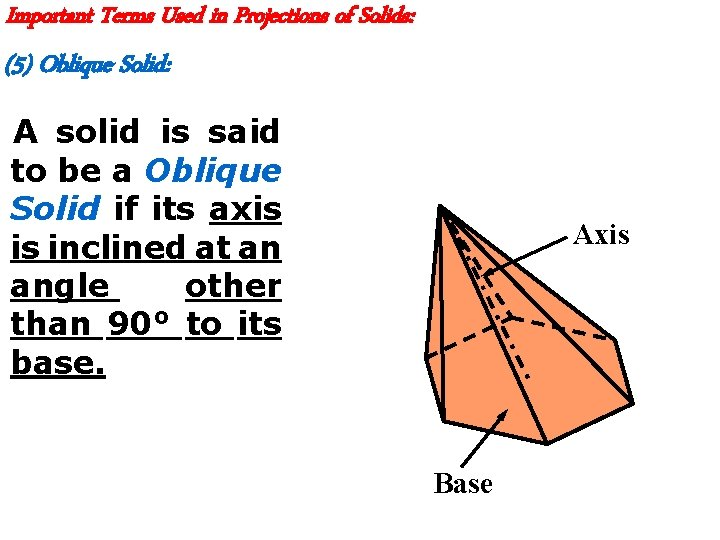Important Terms Used in Projections of Solids: (5) Oblique Solid: A solid is said to be a Oblique Solid if its axis is inclined at an angle other than 90° to its base. Axis BaseImportant Terms Used in Projections of Solids: (6) Regular Solid: A solid is said to be a Regular Solid if all the edges of the base or the end faces of a solid are equal in length and form regular plane figures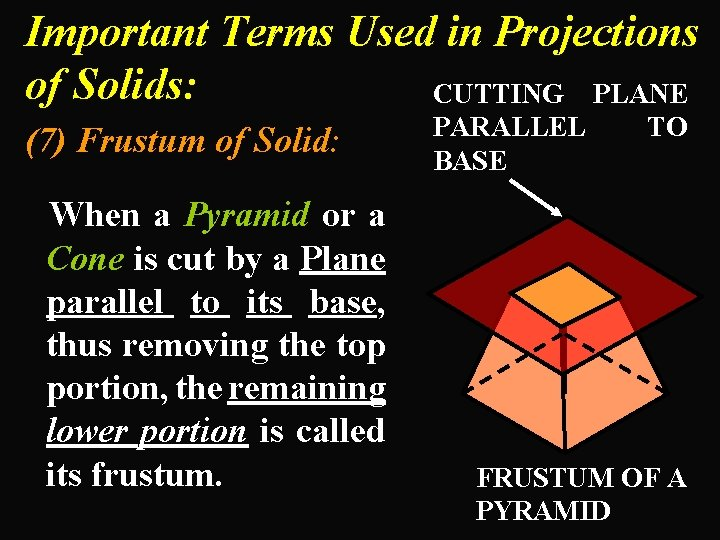Important Terms Used in Projections of Solids: CUTTING PLANE (7) Frustum of Solid: When a Pyramid or a Cone is cut by a Plane parallel to its base, thus removing the top portion, the remaining lower portion is called its frustum. PARALLEL BASE TO FRUSTUM OF A PYRAMID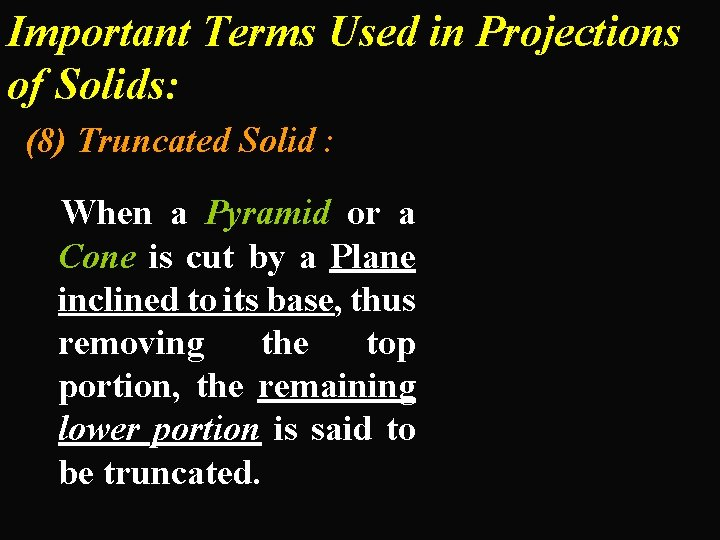Important Terms Used in Projections of Solids: (8) Truncated Solid : When a Pyramid or a Cone is cut by a Plane inclined to its base, thus removing the top portion, the remaining lower portion is said to be truncated.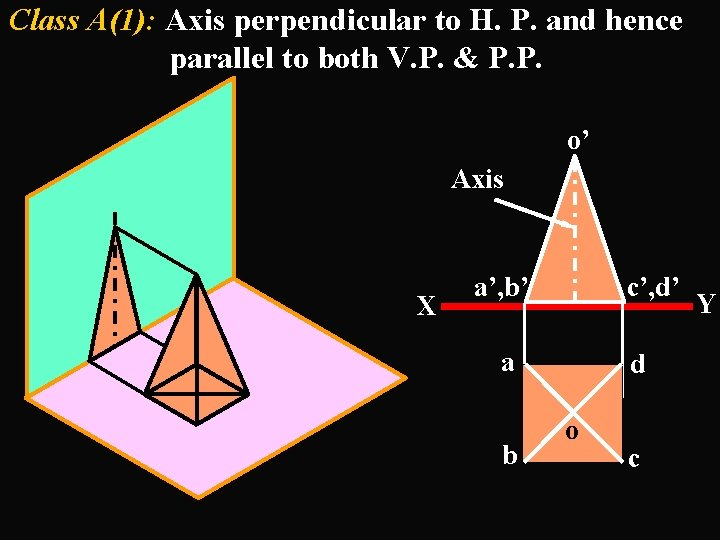Class A(1): Axis perpendicular to H. P. and hence parallel to both V. P. & P. P. o’ Axis X a’, b’ c’, d’ a b d o c Y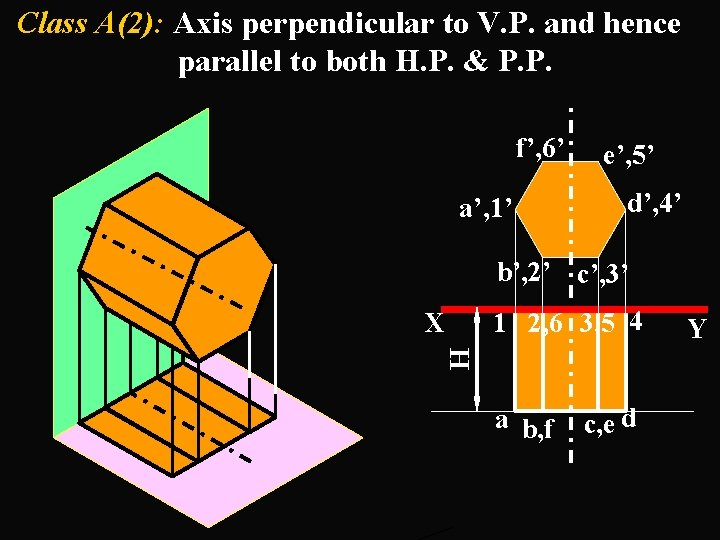Class A(2): Axis perpendicular to V. P. and hence parallel to both H. P. & P. P. f’, 6’ a’, 1’ e’, 5’ d’, 4’ b’, 2’ c’, 3’ 1 2, 6 3, 5 4 H X a b, f c, e d Y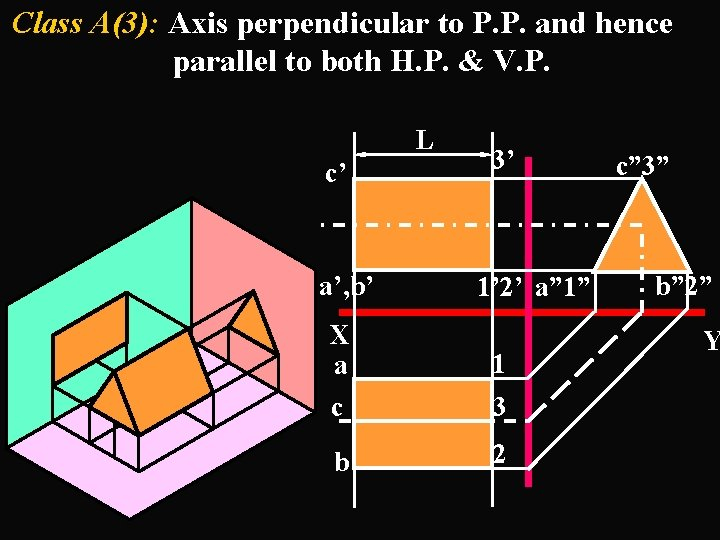Class A(3): Axis perpendicular to P. P. and hence parallel to both H. P. & V. P. L c’ a’, b’ 3’ 1’ 2’ a” 1” X a 1 c 3 b 2 c” 3” b” 2” Y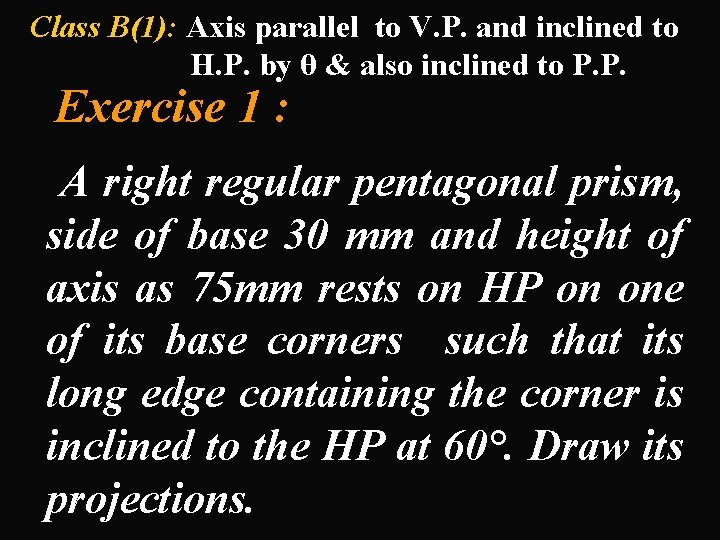Class B(1): Axis parallel to V. P. and inclined to H. P. by θ & also inclined to P. P. Exercise 1 : A right regular pentagonal prism, side of base 30 mm and height of axis as 75 mm rests on HP on one of its base corners such that its long edge containing the corner is inclined to the HP at 60°. Draw its projections.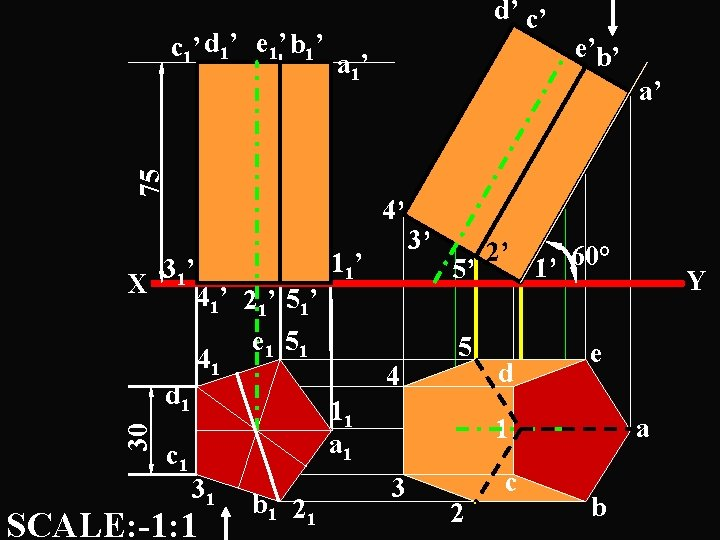a 1’ 75 c 1’ d 1’ e 1’ b 1’ d’ c’ X d 1 30 a’ 4’ 31’ c 1 41’ 21’ 51’ e 1 51 41 11’ 4 3’ 5’ 5 11 a 1 31 SCALE: -1: 1 b 1 21 e’b’ 2’ d 1’ 60° e a 1 3 c 2 Y ba 1’ 75 c 1’ d 1’ e 1’ b 1’ d’ c’ X d 1 30 a’ 4’ 31’ c 1 41’ 21’ 51’ e 1 51 41 11’ 4 3’ 5’ 5 11 a 1 31 SCALE: -1: 1 b 1 21 e’b’ 2’ d 1’ 60° e a 1 3 c 2 Y bExercise 2 : A tetrahedron of 40 mm long edges, rests on HP on one of its edges such that the face containing that edge is inclined to HP at 30° and the same edge is inclined at 45° to VP. Draw the projections of the solid.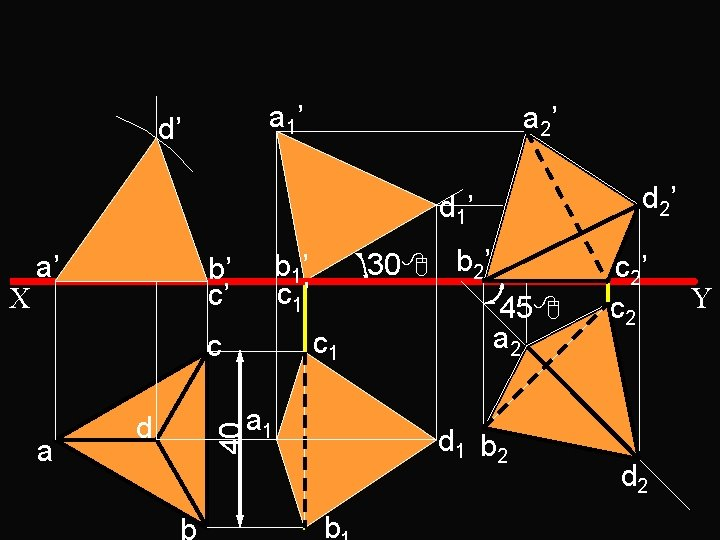a 1’ d’ a 2’ d 1’ c 1 c a 30 b 2’ b 1’ c 1’ b’ c’ a 1 d 40 X a’ b 45 a 2 d 1 b 2 b c 2’ c 2 d 2 Ya 1’ d’ a 2’ d 1’ c 1 c a 30 b 2’ b 1’ c 1’ b’ c’ a 1 d 40 X a’ b 45 a 2 d 1 b 2 b 1 c 2’ c 2 d 2 YExercise 3 : A cone, diameter of base 60 mm and height 70 mm, is resting on HP on the point of periphery of the base. Axis of the cone makes 60 with HP and 30 with the VP. Draw the projections of the cone, when the apex is nearer to the VP.O 1 ’ O’ a 1’ 70 60 X O 2 ’ 60 e 1 ’ e’ a’ b’, h’c’, g’d’, f’ g f h e a b c d a 2’ g 2 ’ g 1 a 1 e 2 g 2 O e 2 ’ O 2 e 1 O a 2 c 1 c 2 ’ 30 Y Locus of. L T. O 2 c 2Exercise 4 : A regular pentagonal prism of 25 mm long edges and axis 70 mm long rests on HP on one of its corner of the base. The slant edge passing through corner makes 45 with HP and the side opposite to the same corner makes 30 with VP. Draw its projections.2’ 1’ 1 a 2 b 4’ a 1’ b 1’ e 1’ c 1’ e’ c’ d’ 5 e a 1 d 4 b 1 c 3 =45 e 1 11 d 1 21 c 1 32’ b 2’ 12’ 52’ 42’ a 2’ e 2’ c 2’ d 1’ d ’ a 2 = 30 2 b 2 Y ` X a’ b’ 3’ 5’ 22’ 11’ 21’ 51’ 31’ 4 ’ 1 51 41 31 c 2 e 2 12 22 d 2 32 52 422’ 1’ 1 a 2 b 4’ a 1’ b 1’ e 1’ c 1’ e’ c’ d’ 5 e a 1 d 4 b 1 c 3 =45 e 1 11 d 1 21 c 1 32’ b 2’ 12’ 52’ 42’ a 2’ e 2’ c 2’ d 1’ d ’ a 2 = 30 2 b 2 Y ` X a’ b’ 3’ 5’ 22’ 11’ 21’ 51’ 31’ 4 ’ 1 51 41 31 c 2 e 2 12 22 d 2 32 52 42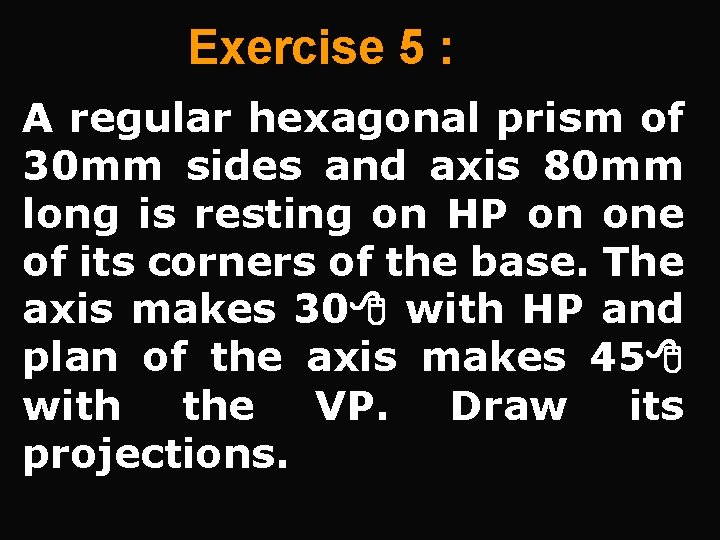Exercise 5 : A regular hexagonal prism of 30 mm sides and axis 80 mm long is resting on HP on one of its corners of the base. The axis makes 30 with HP and plan of the axis makes 45 with the VP. Draw its projections.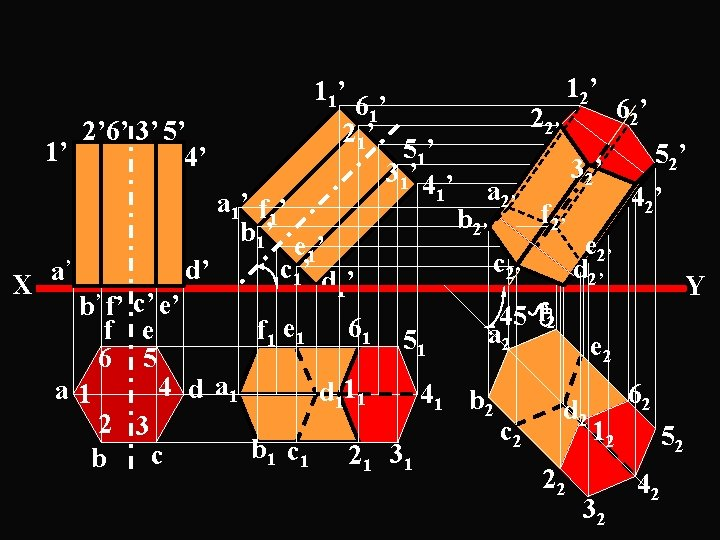2’ 6’ 3’ 5’ 1’ 4’ ’ a X b’ f’ f 6 a 1 2 b 11’ 61’ 21’ 51’ 31’ 4 ’ 1 a 1’ f 1’ b 1’ e 1’ c 1’ d ’ d’ 1 22’ a 2’ b 2’ c 2’ 12’ 32’ f 2’ e 2’ d 2’ 62’ 52’ 42’ c’ e’ f 2 45 61 5 f 1 e a 2 e 2 1 5 4 d a 1 d 111 41 b 2 62 d 2 3 c 2 12 52 b c c 21 31 1 1 22 42 32 YExercise 6 : A square pyramid, side of base 50 mm and height 64 mm, is freely suspended from one of the corners of the base. Draw its projections when vertical plane containing axis makes an angle of 45 with the VP.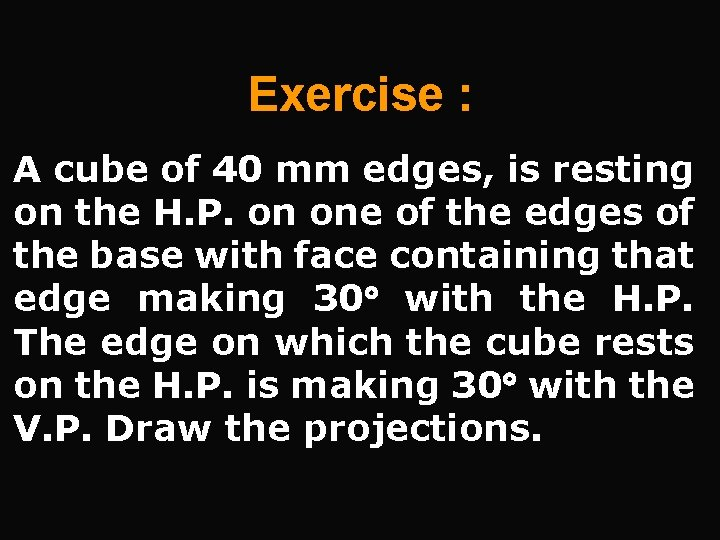Exercise : A cube of 40 mm edges, is resting on the H. P. on one of the edges of the base with face containing that edge making 30 with the H. P. The edge on which the cube rests on the H. P. is making 30 with the V. P. Draw the projections.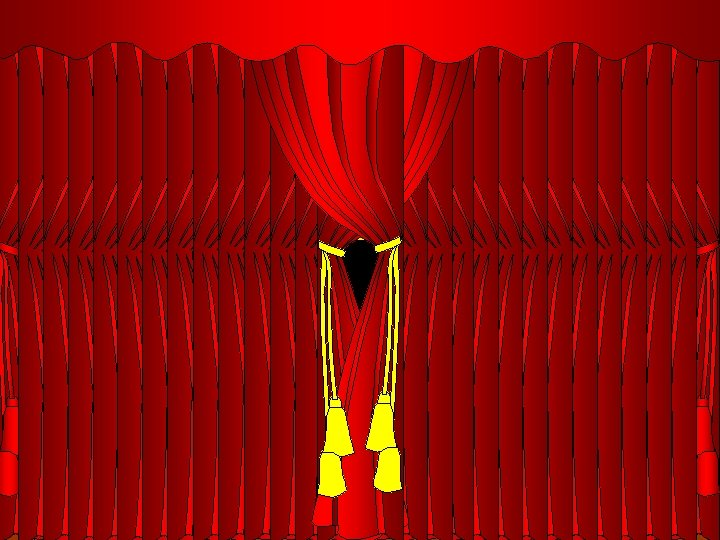PROJECTIONS OF SOLIDS